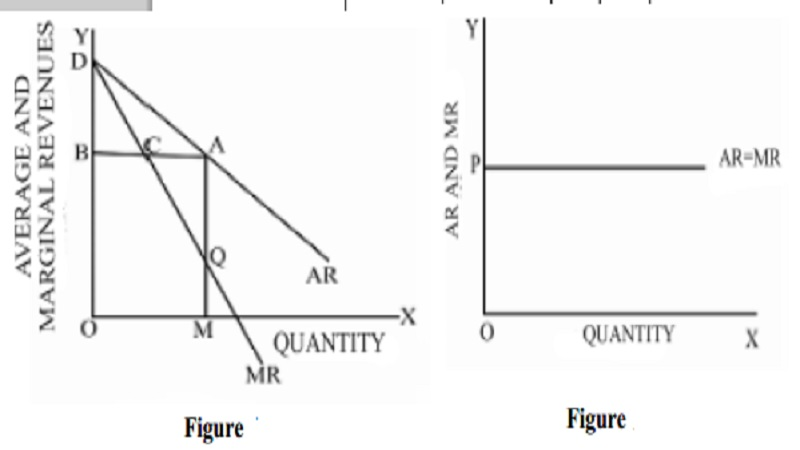Home | | Economics | | Economics | Concepts Of Revenue : Average, Marginal Revenue(AR,MR)

# Concepts Of Revenue : Average, Marginal Revenue(AR,MR)The amount of money, which the firm receives by the sale of its output in the market, is known as its revenue.

## Concepts Of Revenue

The amount of money, which the firm receives by the sale of its output in the market, is known as its revenue.

## Total Revenue

Total Revenue refers to the total amount of money that a firm receives from the sale of its products.

Mathematically TR = PQ where TR = Total Revenue; P = Price; Q = Quantity sold. Suppose a firm sells 1000 units of a product at the price of Rs 10 each, the total revenue will be 1000 x Rs 10 = Rs 10,000/-

## Average Revenue

Average revenue is the revenue per unit of the commodity sold. It is calculated by dividing the total revenue by the number of units sold.

AR = TR / Q Where

AR = Average Revenue

TR = Total Revenue

Q = Quantity sold

Eg: Average Revenue = Rs 10 ,000 / 1000  = Rs 100 / −

Thus average revenue means price of the product.

## Marginal Revenue

Marginal Revenue is the addition made to the total revenue by selling one more unit of a commodity.

For example, if 10 units of a product are sold at the price of Rs 15 and 11 units are sold at Rs14/-, the marginal revenue will be:

MRn = TRn - TRn-1

Rs (11x 14) - Rs (10x 15)

Rs 154 - 150

Rs 4/-

## Relationship between AR and MR curves

When the average revenue (price) remains constant, the marginal revenue will also remain constant and will coincide with the average revenue.

Table Constant AR and MR

No.of units Price or AR          TR    MR

Sold  (Rs)   (Rs)   Rs)

1        10      10      10

2        10      20      10

3        10      30      10

4        10      40      10

5        10      50      10

6        10      60      10

A firm can sell large quantities only at lower prices. In that case, the average revenue (price) of the product falls. When AR falls MR will also fall. But fall in MR will be more than the fall in the AR. Hence the marginal revenue curve will lie below the average revenue curve .

Table Downward sloping AR and MR

No.of units     Price or AR          TR    MR

Sold      (Rs)   (Rs)   Rs)

1  10      10      10

2  9        18      8

3  8        24      6

4  7        28      4

5  6        30      2

6  5        30      0## Measurement of Profit

A firm's profit may be defined as the difference between its total revenue and its total cost i.e. Profit = Total Revenue - Total Cost

The aim of any firm is to maximise its profit i.e. to maximise the positive difference between the Total Revenue (TR) and Total Cost (TC). At that point the producer will be in equilibrium.

Table Maximising Profits

Output (units)      Total Revenue(Rs)         Total Cost (Rs)    Total Profit(Rs)

1        40      45      -5

2        80      70      10

3        130    90      40

4        175    105    70

5        210    130    80

6        240    155    85

7        265    200    65

8        285    255    30

9        290    270    20

10      300    310    -10

From Table it can be understood the firm will earn maximum profit of Rs 85 when it produces 6 units of output. Thus the firm will be in equilibrium by producing 6 units of output.Study Material, Lecturing Notes, Assignment, Reference, Wiki description explanation, brief detail

Related Topics